# Pushing things pulling things and hitting things are

• Slides: 14* Pushing things, pulling things and hitting things are all ways of starting things moving. They move because you make a force act on them. F- force The unit of force is N. Force has a direction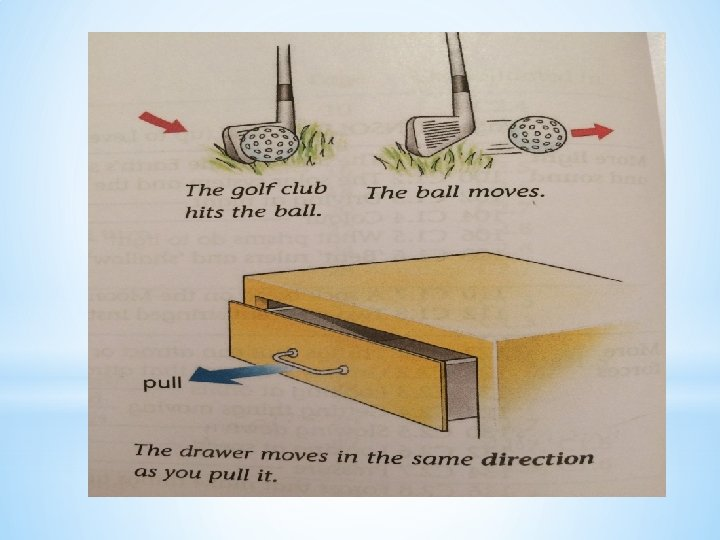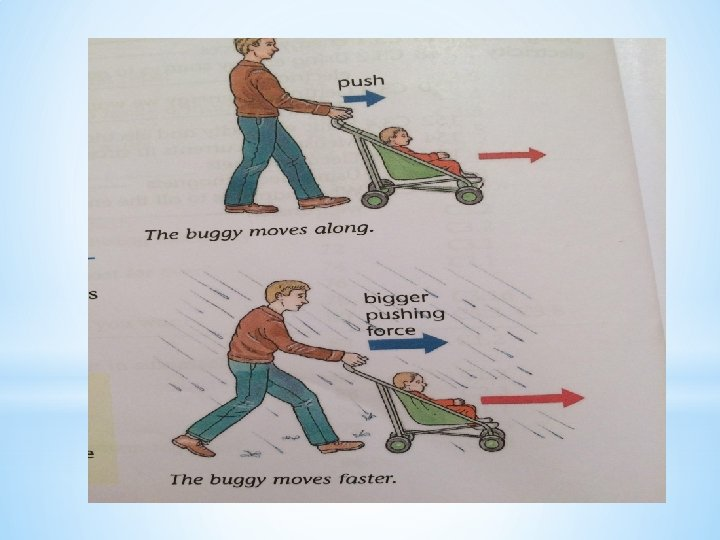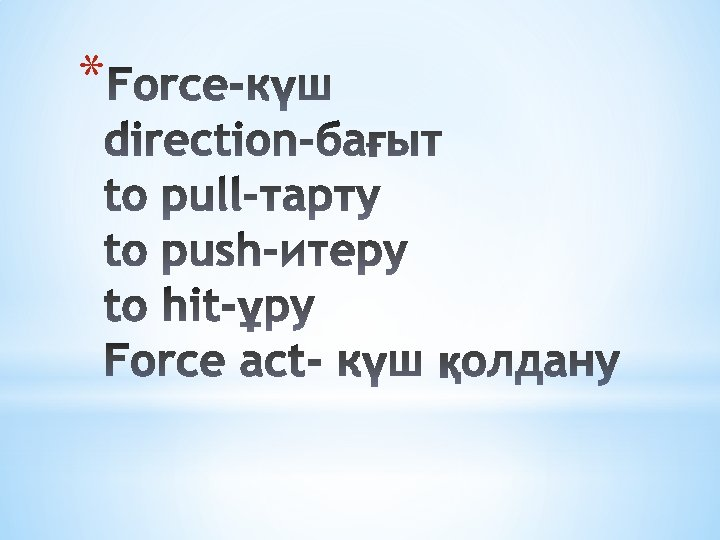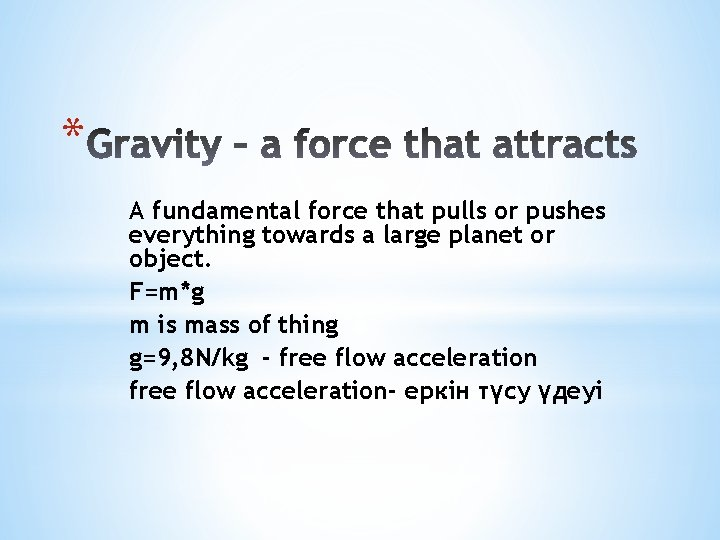* A fundamental force that pulls or pushes everything towards a large planet or object. F=m*g m is mass of thing g=9, 8 N/kg - free flow acceleration- еркін түсу үдеуі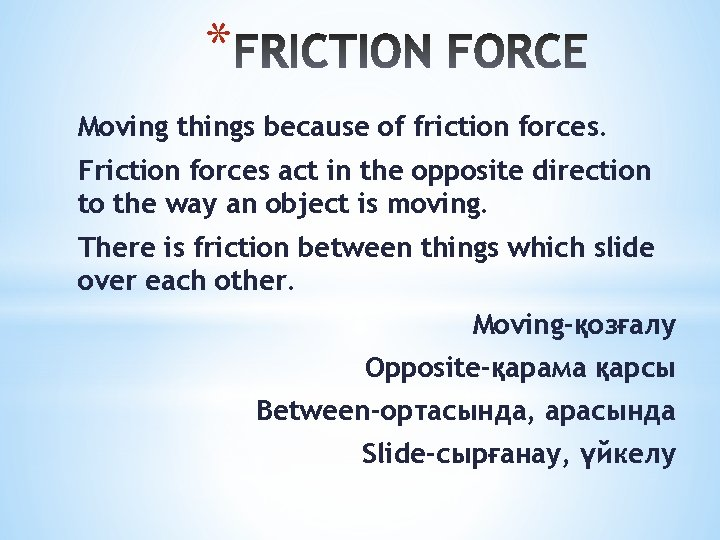* Moving things because of friction forces. Friction forces act in the opposite direction to the way an object is moving. There is friction between things which slide over each other. Moving-қозғалу Opposite-қарама қарсы Between-ортасында, арасында Slide-сырғанау, үйкелу* 1. A force acting on an object makes it _____. 2. The object moves in the same ______as the force. 3. If you want to slow something down, the force must be in the ______direction to the way it is moving. 4. Write the formula of gravity of force. 5. What is the g? 6. The unit of force is___. 7. Moving things slow down because of ________force. la of friction force 8. Write the formula of friction force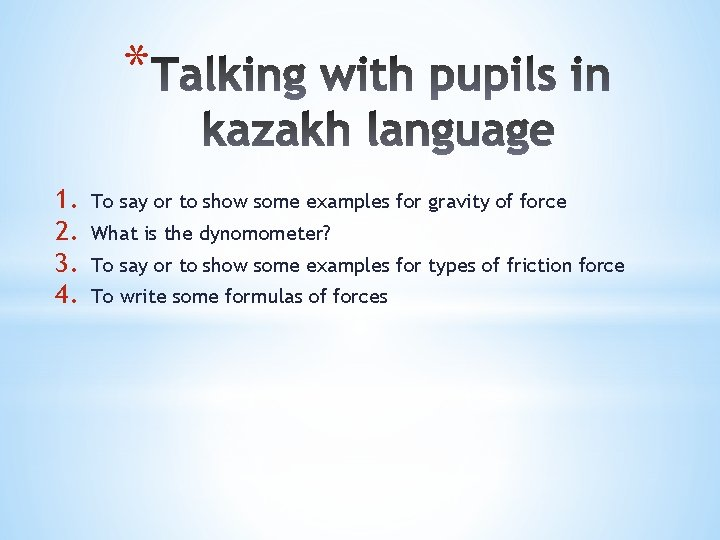* 1. 2. 3. 4. To say or to show some examples for gravity of force What is the dynomometer? To say or to show some examples for types of friction force To write some formulas of forces* 1. 2. 3. 4. 5. 6. 7. 8. F=30 N μ=0, 4 m=12 kg F=210 N m=1000 kg m=120 kg F= 100 N F= 90 N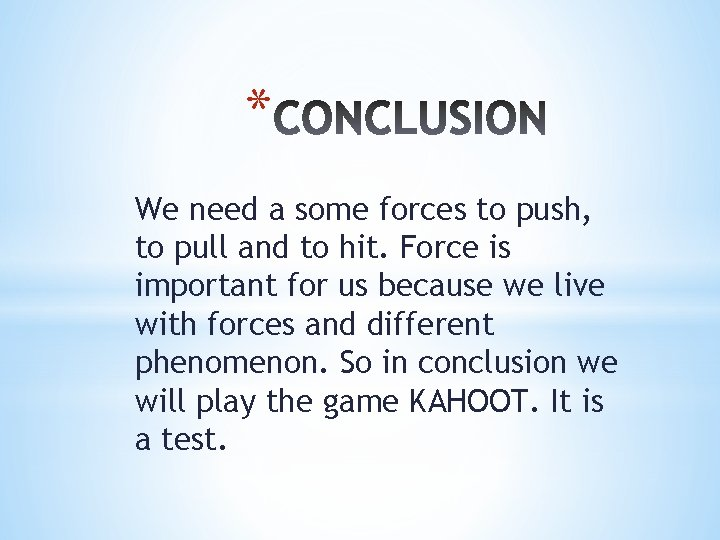* We need a some forces to push, to pull and to hit. Force is important for us because we live with forces and different phenomenon. So in conclusion we will play the game KAHOOT. It is a test.